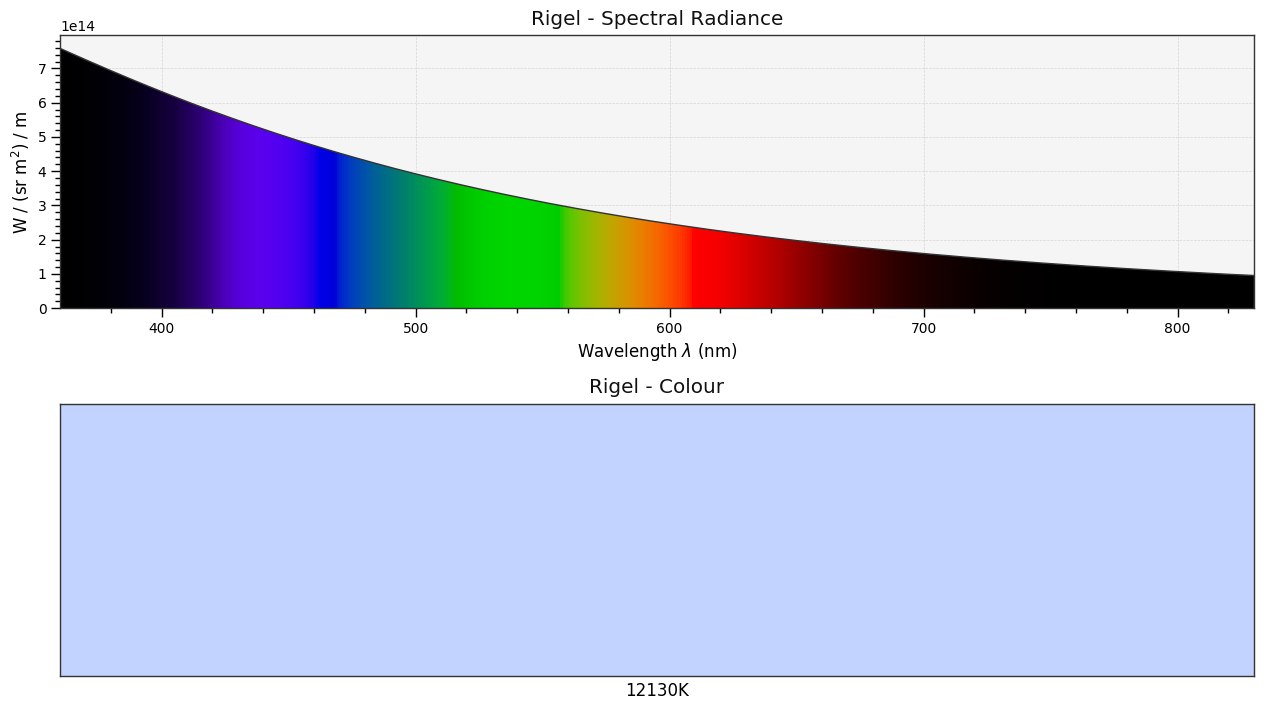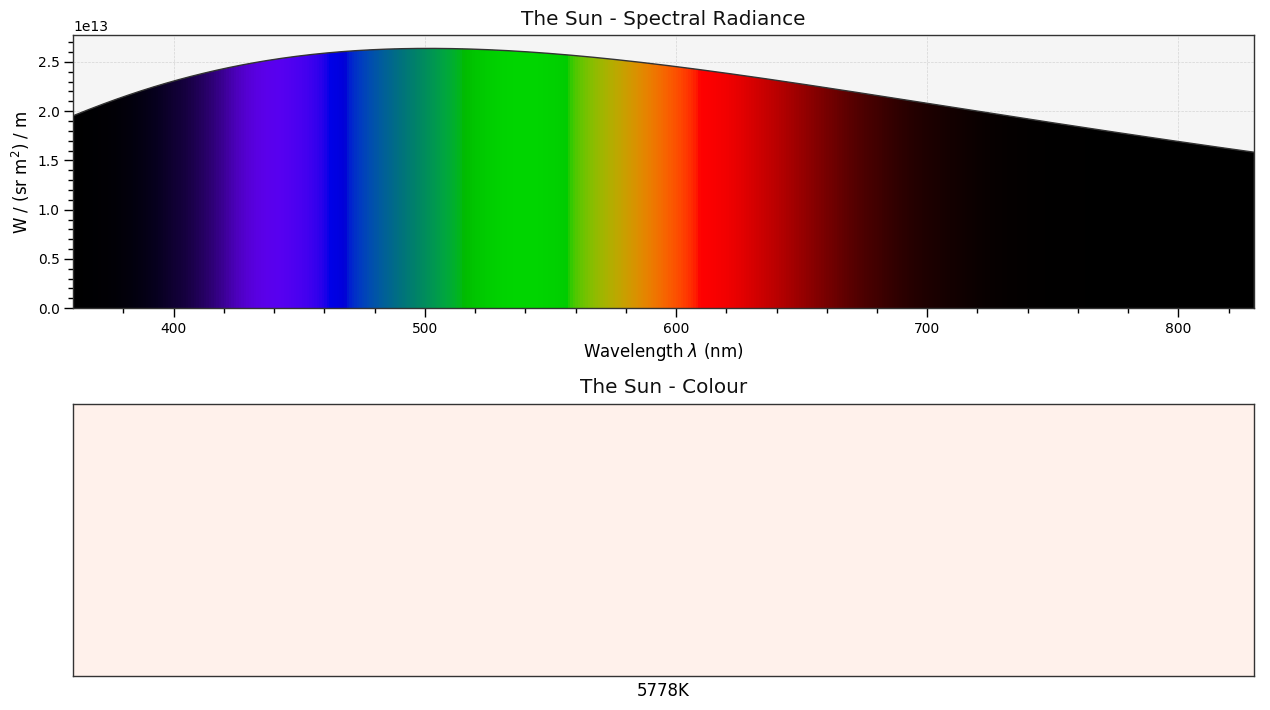Blackbody¶

A blackbody or planckian radiator is an ideal thermal radiator that absorbs completely all incident radiation, whatever the wavelength, the direction of incidence or the polarization. 

Planck's Law¶

The spectral radiance of a blackbody at thermodynamic temperature $T [K]$ in a medium having index of refraction $n$ is given by the Planck's law equation: 

$$\begin{equation} L_{e\lambda}(\lambda,T)=\cfrac{C_1n^{-2}\lambda^{-5}}{\pi}{\Biggl[\exp\biggl(\cfrac{C_2}{n\lambda T}\biggr)-1\Biggr]^{-1}} \end{equation}$$

where \begin{equation} \begin{aligned} C_1&=2\pi hc^2\\ C_2&=\cfrac{hc}{k} \end{aligned} \end{equation}

$h$ is Planck's constant, $c$ is the speed of light in vacuum, $k$ is the Boltzmann constant and $\lambda$ is the wavelength.

As per CIE 015:2004 Colorimetry, 3rd Edition recommendation $C_2$ value when used in colorimetry should be $C_2= 1,4388x10^{-2}mK$ as defined by the International Temperature Scale (ITS-90).

$C_1$ value is given by the Committee on Data for Science and Technology (CODATA) and should be $C_1=3,741771x10^{16}Wm^2$.

In the current CIE 015:2004 Colorimetry, 3rd Edition recommendation, colour temperature and correlated colour temperature are calculated with $n=1$.

Colour implements various blackbody computation related objects in the colour.colorimetry sub-package:

In :
import colour.colorimetry

Note: colour.colorimetry package public API is also available from the colour namespace.

The Planck's law is called using either the colour.planck_law or colour.blackbody_spectral_radiance definitions, they are expecting the wavelength $\lambda$ to be given in nanometers and the temperature $T$ to be given in degree kelvin:

In :
import colour

colour.colorimetry.planck_law(500 * 1e-9, 5500)
Out:
20472701909806.578

Generating the spectral distribution of a blackbody is done using the colour.sd_blackbody definition:

In :
with colour.utilities.suppress_warnings(python_warnings=True):
colour.sd_blackbody(6500, colour.SpectralShape(0, 10000, 10))

With its temperature lowering, the blackbody peak shifts to longer wavelengths while its intensity decreases:

In :
from colour.plotting import *
In :
colour_style();
In :
# Plotting various *blackbodies* spectral distributions.
blackbodies_sds = [colour.sd_blackbody(i, colour.SpectralShape(0, 10000, 10))
for i in range(1000, 15000, 1000)]
with colour.utilities.suppress_warnings(python_warnings=True):
plot_multi_sds(blackbodies_sds,
y_label='W / (sr m$^2$) / m',
use_sds_colours=True,
normalise_sds_colours=True,
legend_location='upper right',
bounding_box=[0, 1000, 0, 2.25e15]);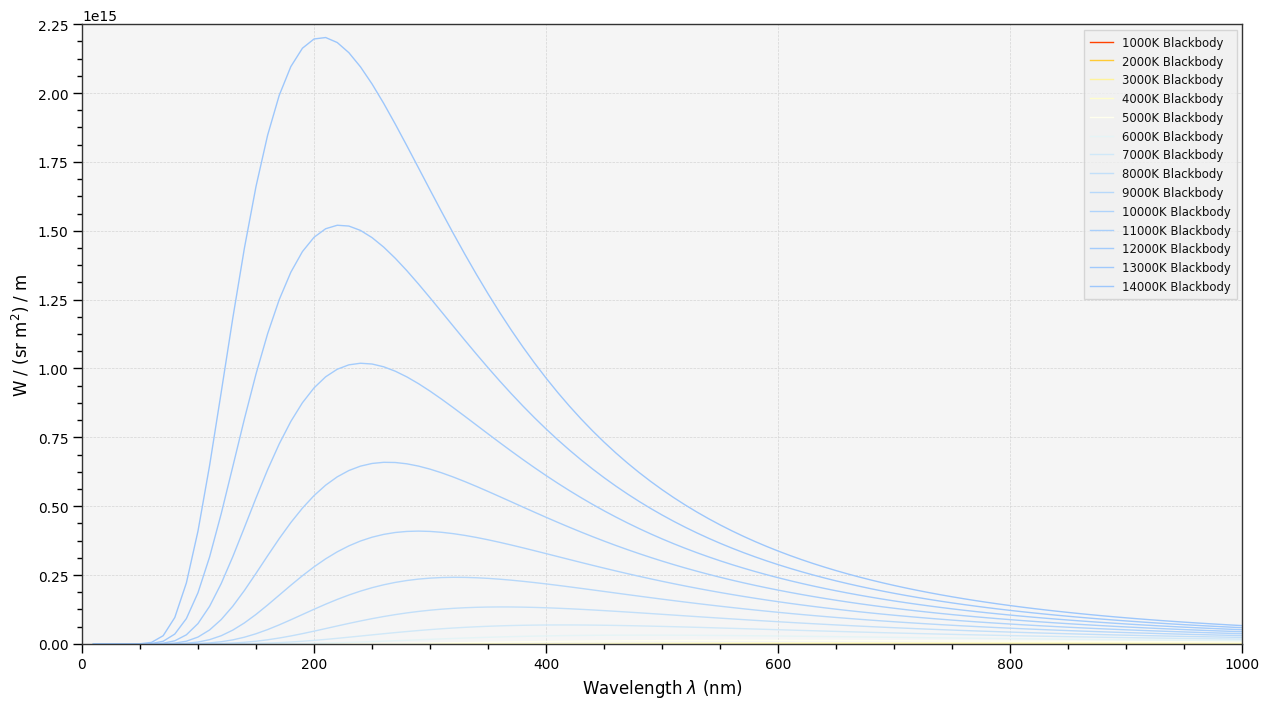Let's plot the blackbody colours from temperature in domain [150, 12500, 50]:

In :
plot_blackbody_colours(colour.SpectralShape(500, 12500, 50));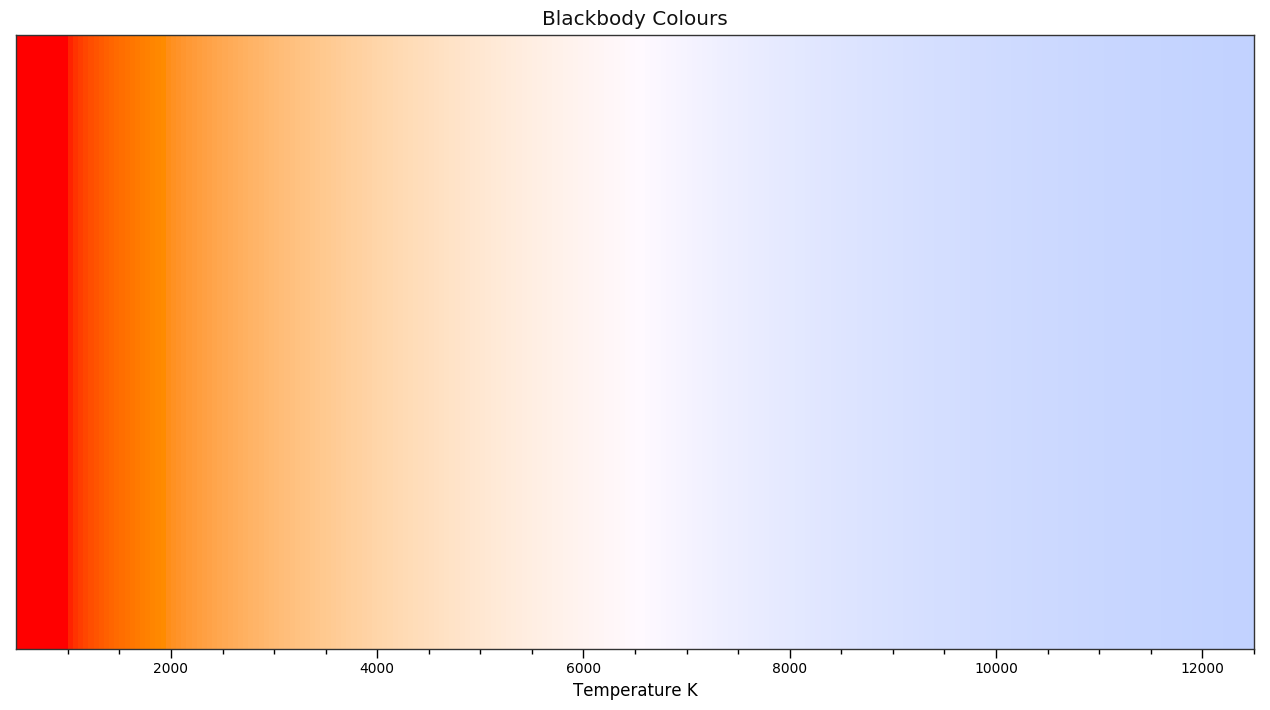Stars Colour¶

Let's compare the extraterrestrial solar spectral irradiance to the blackbody spectral radiance of a thermal radiator with a temperature of 5778 K:

In :
# Comparing theoretical and measured *Sun* spectral distributions.
# Arbitrary ASTMG173_ETR scaling factor calculated with
# :def:colour.sd_to_XYZ definition.
ASTMG173_sd = ASTMG173_ETR.copy() * 1.37905559e+13

blackbody_sd = colour.sd_blackbody(
5778,
ASTMG173_sd.shape)
blackbody_sd.name = 'The Sun - 5778K'

plot_multi_sds([ASTMG173_sd, blackbody_sd],
y_label='W / (sr m$^2$) / m',
legend_location='upper right');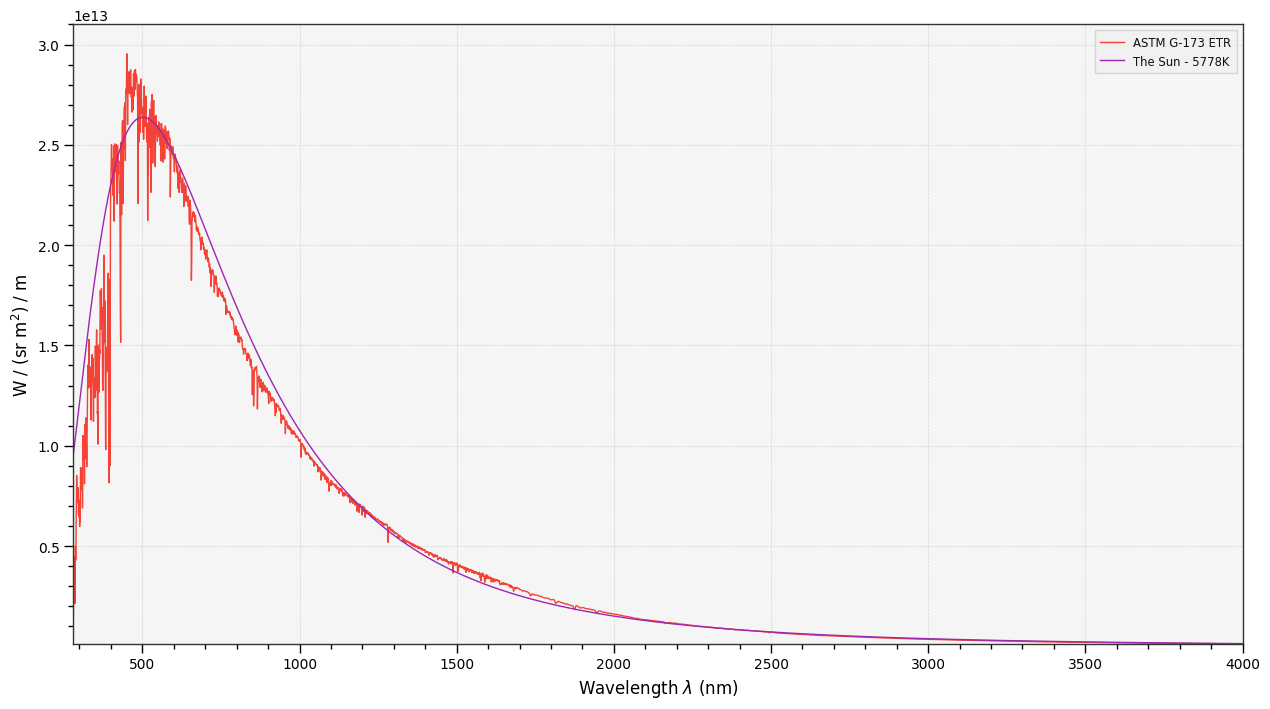As you can see the Sun spectral distribution is very close to the one from a blackbody at similar temperature $T$.

Calculating theoritical colour of any star is possible, for example the VY Canis Majoris red hypergiant in the constellation Canis Major.

In :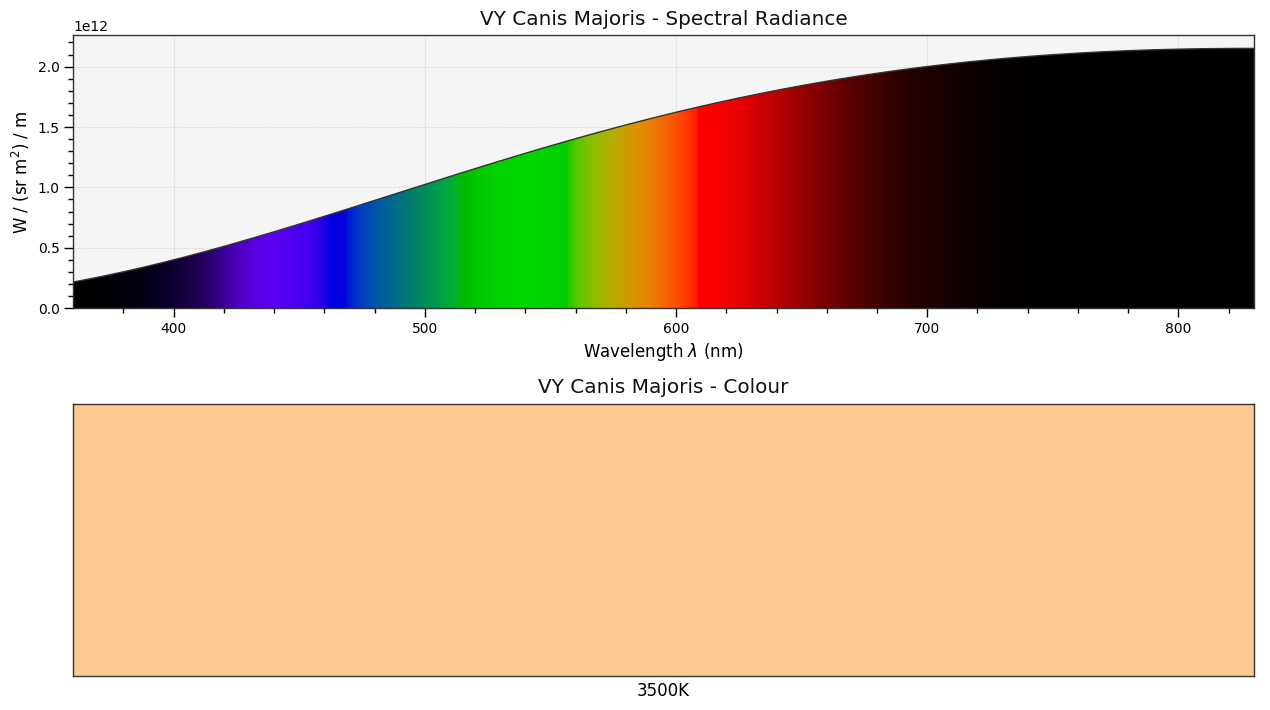Or Rigel the brightest star in the constellation Orion and the seventh brightest star in the night sky.

In :